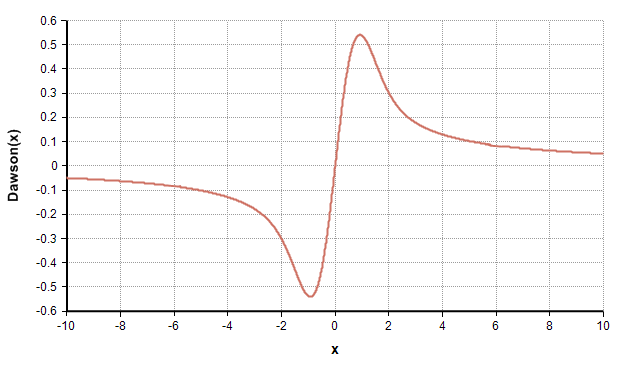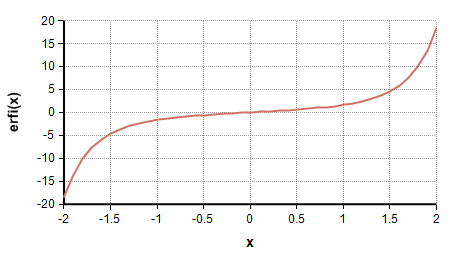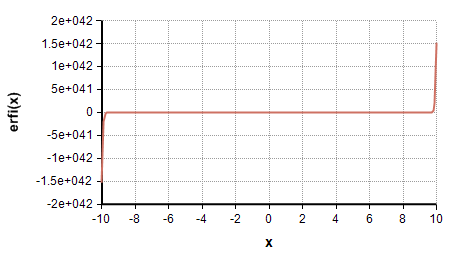# Dawson

## Dawson(x)

The Dawson function, or Dawson Integral, is a function defined on both real numbers and complex numbers, involving the integral of $\displaystyle{ e^{t^2} }$, but scaled by $\displaystyle{ e^{-x^2} }$ so that you don't encounter numeric overflow. It is closely related to the Erf, Faddeeva and CumNormal functions. In mathematical texts, it is often written as $\displaystyle{ F(x) }$ or $\displaystyle{ D_+(x) }$.

The Dawson function is defined as

$\displaystyle{ Dawson(x) = e^{-x^2} \int_0^x e^{t^2} dt }$The $\displaystyle{ e^{t^2} }$ term appears in many mathematical and statistical contexts, but creates numeric difficulties since $\displaystyle{ e^{t^2} }$ overflows the largest double floating point when t ≥ 27. When you encounter this situation, you can often get your computation to succeed, without pushing the numeric limits, by reformulating the calculation in terms of the Dawson function.

## Erfi(x)The Dawson function can be interpreted as a scaled version of the $\displaystyle{ erfi(x) }$ function, which the relationship between the two given as

$\displaystyle{ erfi(x) = {2\over\sqrt\pi} e^{x^2} D(x) }$

The $\displaystyle{ erfi(x) }$ function, which is used occasionally in mathematical publication, is not a built-in function in Analytica; however, if you have an application that uses erfi, you should almost always implement your algorithm using Dawson instead, otherwise you are likely to experience numeric overflow.